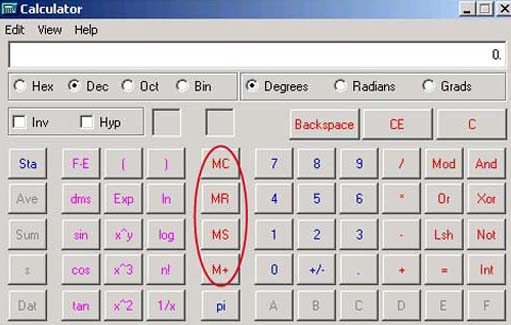Presenting information

Start this free course now. Just create an account and sign in. Enrol and complete the course for a free statement of participation or digital badge if available.

Free course

# 1.1.7 Using the memory buttons

Calculations involving several operations can also be carried out in stages. One way to do this is to use the '=' key part way through the calculation. You can also use the calculator's memory.

The Windows calculator has a number of memory buttons, shown in Figure 2, to help you to perform calculations that have multiple steps.Figure 2 The memory buttons

MS (memory store) stores the displayed number in memory.

MR (memory recall) recalls and displays the number stored in memory, erasing any number that was previously displayed. The number itself is still stored in memory.

M+ (memory +) adds the number displayed to the number stored in memory.

MC (memory clear) deletes the number stored in memory.

For example, let's repeat the fourth calculation in the previous activity: add together 8 and 15 and divide 46 by the result.

1. Enter '8+15 ='. The calculator will display '23'.

2. Then click the 'MS' key; this will insert the 23 into the calculator's memory.

3. Use the 'C' key to clear the calculator's display.

4. Enter '46 /' and press 'MR' to recall the 23 from memory.

5. Enter '=' to display the result.

## Activity 3 (self-assessment)

Try the following calculation using the memory keys. Before you start, use the MC key to clear the calculator's memory, and the 'C' key to clear the display.

Add 63 to 39 then subtract the result from 181.

1. Enter '63+39 ='. The calculator will display '102'.

2. Then click the 'MS' key; this will insert the 102 into the calculator's memory.

3. Enter '181 −' and press 'MR' to recall the 102 from memory.

4. Enter '=' to display the result, which should be 79.

T175_7